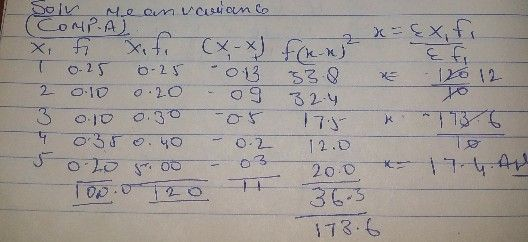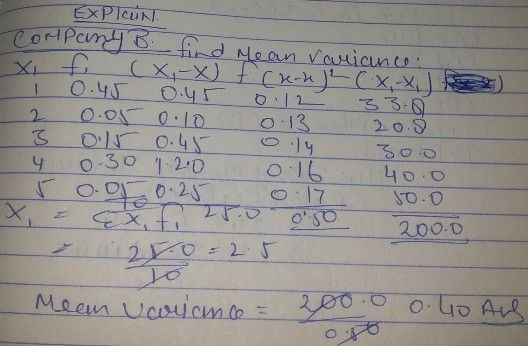Symbol
ProblemFind the mean and vananc. Nw $∴$ CompanyA $2$ $1$ $3$ $4$ $5$ $\left(y\right)1$ $0.25$ $0-16$ $0.10$ $0.3\sqrt{}$ $0.20$ Compeny $B$ $N$ $\dfrac {2} {0^{.05}}$ $3$ $P\left(y$ $0-45|0.05|0.15$ $4$ $030^{\right)^{\sqrt{} }}-05$